# Transformation of Functions

By applying certain transformations to the graph of a given function we can obtain new functions. This will give the ability to sketch the graphs of many functions quickly based on the old one. It will also be easier to write equations for given graphs.

Translations

Suppose ${a}>{0}$. To obtain the graph of

1. ${y}={f{{\left({x}\right)}}}+{a}$, shift the graph of ${f{{\left({x}\right)}}}$ ${a}$ units upward.
2. ${y}={f{{\left({x}\right)}}}-{a}$, shift the graph of ${f{{\left({x}\right)}}}$ ${a}$ units downward.
3. ${y}={f{{\left({x}-{a}\right)}}}$, shift the graph of ${f{{\left({x}\right)}}}$ ${a}$ units to the right.
4. ${y}={f{{\left({x}+{a}\right)}}}$, shift the graph of ${f{{\left({x}\right)}}}$ ${a}$ units to the left.

Example 1. Draw following graphs of functions: ${y}=\sqrt{{{x}}}$, ${y}=\sqrt{{{x}}}+{5}$, ${y}=\sqrt{{{x}}}-{3}$, ${y}=\sqrt{{{x}-{3}}}$, ${y}=\sqrt{{{x}+{2}}}$.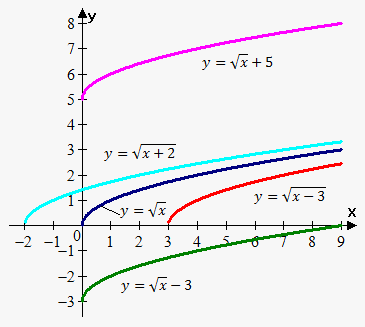We first draw ${y}=\sqrt{{{x}}}$, then we move it 5 units upward to obtain ${y}=\sqrt{{{x}}}+{5}$.

Again we move ${y}=\sqrt{{{x}}}$ 3 units downward to obtain ${y}=\sqrt{{{x}}}-{3}$.

${y}=\sqrt{{{x}-{3}}}$ is obtained from ${y}=\sqrt{{{x}}}$ by moving it 3 units to the right.

${y}=\sqrt{{{x}+{2}}}$ is obtained from ${y}=\sqrt{{{x}}}$ by moving it 2 units to the left.

Stretching/Compressing and Reflection

Suppose ${b}>{1}$. To obtain the graph of

1. ${y}={b}{f{{\left({x}\right)}}}$, stretch the graph of ${y}={f{{\left({x}\right)}}}$ vertically by a factor of ${b}$.
2. ${y}=\frac{{1}}{{b}}{f{{\left({x}\right)}}}$, compress the graph of ${y}={f{{\left({x}\right)}}}$ vertically by a factor of ${b}$.
3. ${y}={f{{\left({b}{x}\right)}}}$, compress the graph of ${y}={f{{\left({x}\right)}}}$ horizontally by a factor of ${b}$.
4. ${y}={f{{\left(\frac{{x}}{{b}}\right)}}}$, stretch the graph of ${y}={f{{\left({x}\right)}}}$ horizontally by a factor of ${b}$.
5. ${y}=-{f{{\left({x}\right)}}}$, reflect the graph of ${y}={f{{\left({x}\right)}}}$ about the x-axis.
6. ${y}={f{{\left(-{x}\right)}}}$, reflect the graph of ${y}={f{{\left({x}\right)}}}$ about the y-axis.

Example 2. Draw following graphs of trigonometric functions: ${y}={\sin{{\left({x}\right)}}}$, ${y}={2}{\sin{{\left({x}\right)}}}$, ${y}=\frac{{1}}{{4}}{\sin{{\left({x}\right)}}}$.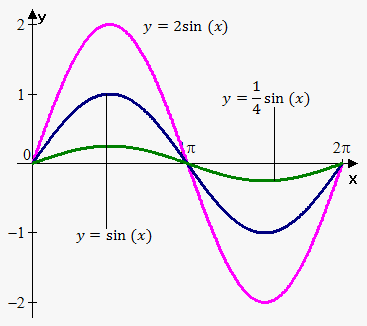We first draw ${y}={\sin{{\left({x}\right)}}}$.

Then stretch it vertically by a factor of 2 to obtain ${y}={2}{\sin{{\left({x}\right)}}}$. Again we compress ${y}={\sin{{\left({x}\right)}}}$ vertically by a factor of 4 to obtain ${y}=\frac{{1}}{{4}}{\sin{{\left({x}\right)}}}$.

Note, that we have drawn graphs only on interval ${\left[{0},{2}\pi\right]}$ because sine is periodic function with period ${2}\pi$.

Example 3. Draw following graphs of trigonometric functions: ${y}={\sin{{\left({x}\right)}}}$, ${y}={\sin{{\left({2}{x}\right)}}}$, ${y}={\sin{{\left(\frac{{1}}{{3}}{x}\right)}}}$.

We first draw ${y}={\sin{{\left({x}\right)}}}$.

Then compress it horizontally by a factor of 2 to obtain ${y}={\sin{{\left({2}{x}\right)}}}$.

Again we stretch ${y}={\sin{{\left({x}\right)}}}$ horizontally by a factor of 3 to obtain ${y}={\sin{{\left(\frac{{1}}{{3}}{x}\right)}}}$.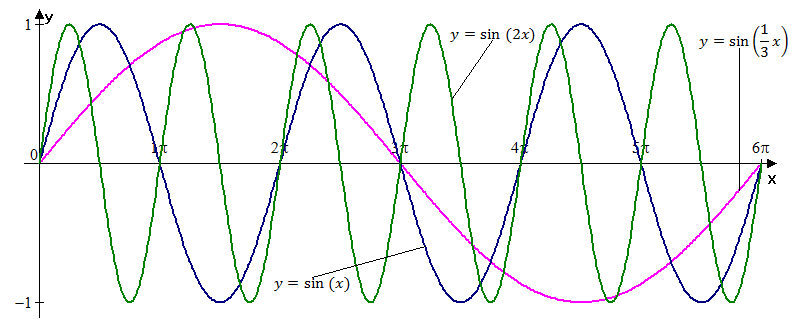Example 4. Draw following graphs of functions: ${y}=\sqrt{{{x}}}$,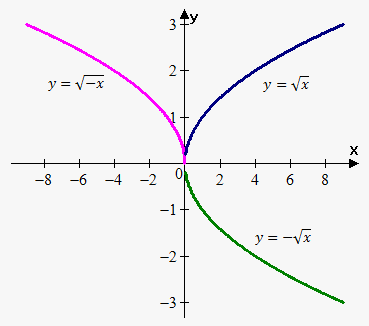${y}=-\sqrt{{{x}}}$, ${y}=\sqrt{{-{x}}}$.

We first draw ${y}=\sqrt{{{x}}}$ then reflect it about x-axis to obtain ${y}=-\sqrt{{{x}}}$.

Again we reflect ${y}=\sqrt{{{x}}}$ about y-axis to obtain ${y}=\sqrt{{-{x}}}$.

Note that domain of ${y}=\sqrt{{{x}}}$ and ${y}=-\sqrt{{{x}}}$ is ${x}\ge{0}$, while domain of ${y}=-\sqrt{{{x}}}$ is ${x}\le{0}$.

Example 5. How can we obtain graph of the function ${y}={1}-{2}\sqrt{{{x}}}$ from graph of function ${y}=\sqrt{{{x}}}$?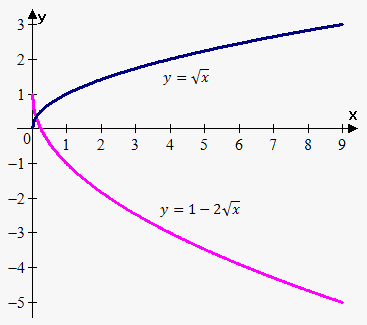We first draw graph of the function ${y}=\sqrt{{{x}}}$.

Then we stretch it vertically to obtain ${y}={2}\sqrt{{{x}}}$.

Then we reflect result about x-axis to obtain ${y}=-{2}\sqrt{{{x}}}$.

Finally, we obtain ${y}={1}-{2}\sqrt{{{x}}}$ by shifting ${y}=-{2}\sqrt{{{x}}}$ 1 unit upward.

Alternatively we could reflect ${y}=\sqrt{{{x}}}$ about x-axis, then stretch result vertically by a factor of 2 and then shift ${y}=-{2}\sqrt{{{x}}}$ 1 unit upward.

Example 6. Draw graph of the function ${y}={2}{{x}}^{{2}}+{8}{x}+{5}$.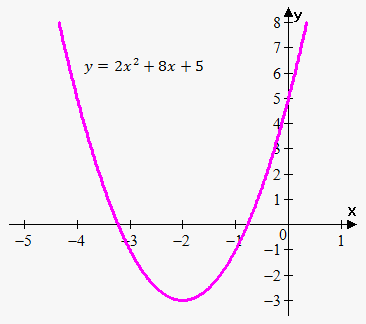First we complete a square: ${y}={2}{{x}}^{{2}}+{8}{x}+{8}-{3}=$

$={2}{\left({{x}}^{{2}}+{4}{x}+{4}\right)}-{3}={2}{{\left({x}+{2}\right)}}^{{2}}-{3}$.

First of all, let's draw graph of the function ${y}={{x}}^{{2}}$.

Then shift it 2 units to the left.

Then stretch result vertically by a factor of 2.

And finally shift it 3 units downward.

Finalt graph is shown on the picture.